# 5.2   Turing Machines

This section under major construction.

## Turing machine.

The Turing machine is one of the most beautiful and intriguing intellectual discoveries of the 20th century. Turing machine is a simple and useful abstract model of computation (and digital computers) that is general enough to embody any computer program. It forms the foundation of theoretical computer science. Because of its simple description and behavior, it is amenable to mathematical analysis. This analysis has led to a deeper understanding of digital computers and computation, including the revelation that there are some computational problems that cannot be solved on computers at all, no matter how fast the processor, or how much memory is available.

Turing machine simulator. This is a graphical Turing machine simulator that was written in Java by Tom Ventimiglia under the supervision of Bob Sedgewick and Kevin Wayne.

• Executable jar (turing.jar). To execute, type java -jar turing.jar from the command line.

• OS X app (Turing.zip). To execute, double click Turing.zip file to unzip. Double click Turing.app to launch.
You are welcome to inspect and modify the source code for your own use.

Components.  Alan Turing sought to describe the most primitive model of a mechanical device that had the same basic capabilities as a human "computer." In his epoch making 1936 paper, Alan Turing introduced an abstract machine, which would later come to be known as a Turing machine. The machine consists of the following components:

• The ticker-tape stores the input, the intermediate results, and the output. The tape is one arbitrarily long strip, divided into cells. Each cell stores one of a finite alphabet of symbols. In the example below, we use a 4 character alphabet consisting of 0, 1, A, X, and #.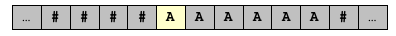• The tape head of the Turing machine scans the tape one cell at a time. We refer to the cell being scanned as the active cell and the symbol it contains as the input symbol. At each time step, the tape head reads the input symbol, and leaves it either unchanged or overwrites it with a new symbol. At the end of each time step, the tape head moves one position to the left or right. We highlight the active cell in yellow. In the example below, the A is replaced with an X and the tape head moves one cell to the left.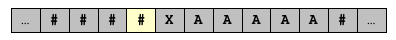• The control unit is the analog of the CPU in modern day microprocessors. It consists of a state transition diagram, which is a finite table of instructions that specifies exactly what action the machine takes at each step. Each state represents one of the possible configurations of the machine. Depending on its current state and input symbol, the Turing machine overwrites the input symbol with a new symbol and moves to a new state. Each transition connects one state, say s, to another state, say t, and is labeled with two symbols, say A and X: this means that if the Turing machine is in state s and the input symbol is A, then it overwrite the A with an X and transitions to state t. Each state is labeled with one of five designations: L (left), R (right), Y (yes), N (no), or H (halt). Upon entering a state, the Turing machine either moves its tape head or halts according to the state's designation. Below is an illustration of the state transition diagram for a machine with four states.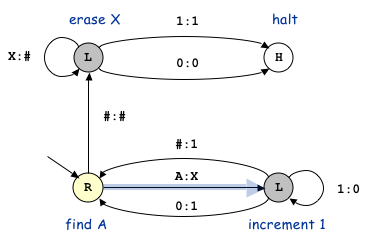Execution.  Initially the Turing machine starts in one distinguished state called the start state, and the tape head points to one distinguished cell called the start cell. There is at most one possible transition corresponding to each combination of state and input symbol; thus, the actions of the machine are completely determined in advance. (If there is no possible transition from a state with some input symbol, then the Turing machine remains in the same state and does not overwrite the input symbol.) Each step in a Turing machine proceeds as follows:

• Read the input symbol from the active cell.
• Look up the transition rule associated with the current state and input symbol.
• Overwrite the input symbol with the new symbol.
• Change the current state according to the transition rule.
• Shift the tape head one cell to the left or right, according to the new state's designation.

These steps are repeated until the current state is labeled H for halt, Y (in which case the machine answers yes) or N (in which case the machine answers no). It is possible that the machine runs forever without ever reaching one of these terminating states.

Computation must allow repetitive actions - do action A over and over until a certain condition is met. This amounts to staying in a state (and moving the tape head left or right) until a certain condition is met. Computation must also allow adaptive actions - if a certain condition is met, do action A; otherwise do action B. This is captured by state transitions according to the contents of the tape head at a particular location.

An example: unary to binary conversion.   We consider the 4 state Turing machine illustrated below. The current state and input symbol are highlighted in yellow. We trace its execution.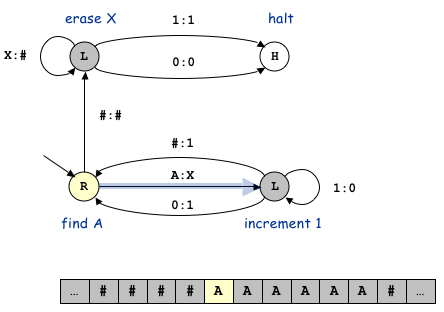Since the input symbol is A, the Turing machine follows the appropriate transition arrow leaving the current state - the one labeled A : X. The Turing machine overwrites the input symbol with an X, changes state to the bottom right state, and moves the tape head one position to the left (since the new state is labeled with L). The illustration below shows the Turing machine at the end of this first step.Since the input symbol is now #, the Turing machine follows the appropriate transition arrow leaving the current state -- the one labeled # : 1. This overwrites the current cell with a 1, changes the state back to the bottom left state, and moves the tape head one position to the right (since the new state is labeled with R).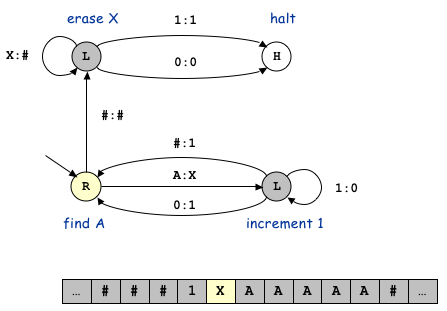Here are the contents of the tape after the next several steps.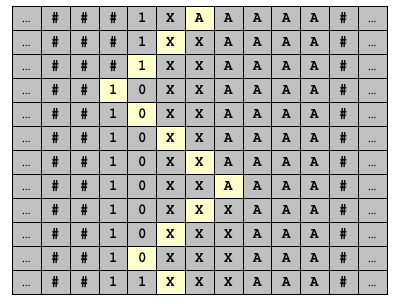(Errata: in the fourth row, the highlighted cell should contain a # instead of a 1.)

Once all of the As are overwritten with Xs, the Turing machine erases all of the Xs (overwriting them with #s).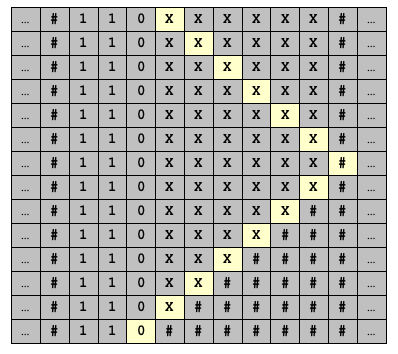What it does and why it works.   The Turing machine described above converts from unary to binary. That is, if the input consists of n consecutive A's, then the Turing machine prints the number n in binary to the left of sequence of A's (and overwrites the A's with X's). In the example above, the input consists of 6 A's and the Turing machine writes the binary number 110 to the tape.

To describe how this is accomplished, we first review an algorithm for incrementing a binary integer by 1: scan the bits from right to left, changing 1's to 0's until you see a 0. Then change the 0 to a 1.

The Turing machine repeatedly knocks off one A at a time and increments the binary number. Our Turing machine mimics this strategy. The initial state seeks out the next A, overwrites it with an X, and then transitions to the Increment state. The Increment state increments the binary integer by one (leaving the X's alone, changing 1's to 0's, until seeing a 0 or #, which it changes to a 1), and then transitions back to the Initial state. When all of the A's are overwritten with X's, the Cleanup state replaces all of the X's with #'s, and the transitions to the Halt state.## Turing machine implementation in Java.

We encapsulate each of the main Turing machine components (tape, transition, control) using good OOP principles.

• The tape. Program Tape.java is an ADT that represents an unbounded Turing machine tape. It supports the following operations: move tape head left, move tape head right, read the symbol in the current cell, and write a symbol to the current cell. To implement it, we use two stacks (one to store all of the symbols to the left of the tape head, and one to the right). To print out the contents of the tape, we print out the reverse of the first stack, the current element, then the second stack.

• The states. Each state has a name and a type (halt, left, right, accept, or reject).

• The transitions. Each transition has the name of the initial state, the name of the final state, and the symbol to be written to the tape.

• The Turing machine. We implement a Turing machine as a tape, a symbol table of states, and a symbol table of transitions.

Non-terminating Turing machines. From a theoretical standpoint, we are primarily concerned with machines that perform finite computations and then halt. However, many practical applications involve programs that are designed never to terminate (operating system, air traffic control system, nuclear reactor control system) or produce an infinite amount of output (web browser, program that computes the digits of π = 3.1415...). The Turing machine model of computation extends to handle such non-terminating situations as well.

Turing machines connect physics and mathematics (Turing's original motivation, thermodynamics of computation).

#### Exercises

1. What does the following Turing machine do when started with the given tape ...
3. Binary counter.
4. Binary palindrome.
5. Unary multiplication.
6. Equal number of a's and b's.
7. Multiple of 3 or 7.
8. Balanced parentheses.
9. Power-of-2.
10. String compare.
11. Unary-to-binary. How many steps does the 3-state unary-to-binary Turing machine make to convert N to binary? Answer: proportional to N^2.
12. Unary-to-binary. Design a 6-state unary-to-binary Turing machine that converts the unary number N to binary in time proportional to N log N. Hint: cross out every other A. If the number of A's is odd, write a 1; otherwise write a 0. Repeat with the uncrossed out A's remaining.13. Swap two cells on a Turing machine. Use the state to encode the temporary symbol.
14. Hex-to-binary. Design a Turing machine that converts from hexadecimal to binary.
15. Comparator. Create a Turing machine that takes as input two binary integers, separate by a # character, and accepts the input if the first string is strictly less than the second. How many steps does the Turing machine in the previous question take to compare two N-bit integers? (Each step is one move of the tape head.)
16. Efficient comparator. Create a comparator that runs in time polynomial in N.
17. Bitwise OR. Create a Turing machine that computes the bitwise OR of its two binary inputs of length N.

#### Creative Exercises

1. Doubling. Write a Turing machine that transform an input consisting of k consecutive 1's to an input that consists of 2k consecutive 1's. (unary multiplication by 2) Hint: write two 1's on the left, and delete one 1 on the right.
2. Copying. Write a Turing machine that transform an input consisting of 0's and 1's instead two copies of the original input, separated by the symbol #.
3. Langtons Ant. Write a program LangtonsAnt.java that simulates a two dimensional Turing machine known as Langton's Ant, and animate the results using Turtle graphics.
4. Turmites. Create some other two dimensional Turing machines or Turmites that produce interesting patterns.
5. Turing tape. Write a program Tape.java that implement a one-dimensional Turing tape. The tape consists of a sequence of cells, each of which stores an integer (that is initialized to 0). At any instant, there is a tape head which points to one of the cells. Support the following interface methods: moveLeft() to move the tape head one cell to the left, moveRight() to move the tape head one cell to the right, look() to return the contents of the active cell, and write(int a) to change the contents of the active cell to a. Hint: use an int for the active cell, and two stacks for the left and right parts of the tape.
6. Turing machine simulator. Write a program TuringMachine.java that simulates a Turing machine. Design your program as follows: Tape.java, State.java, Transition.java.
7. Collatz Turing machine. Design a Turing machine that takes as input the binary representation of a binary integer and repeatedly divides it by 2 (if even) or multiplies by 3 and adds 1 (if odd) until it equals 1. Famous open conjecture that this machine will terminates for inputs.